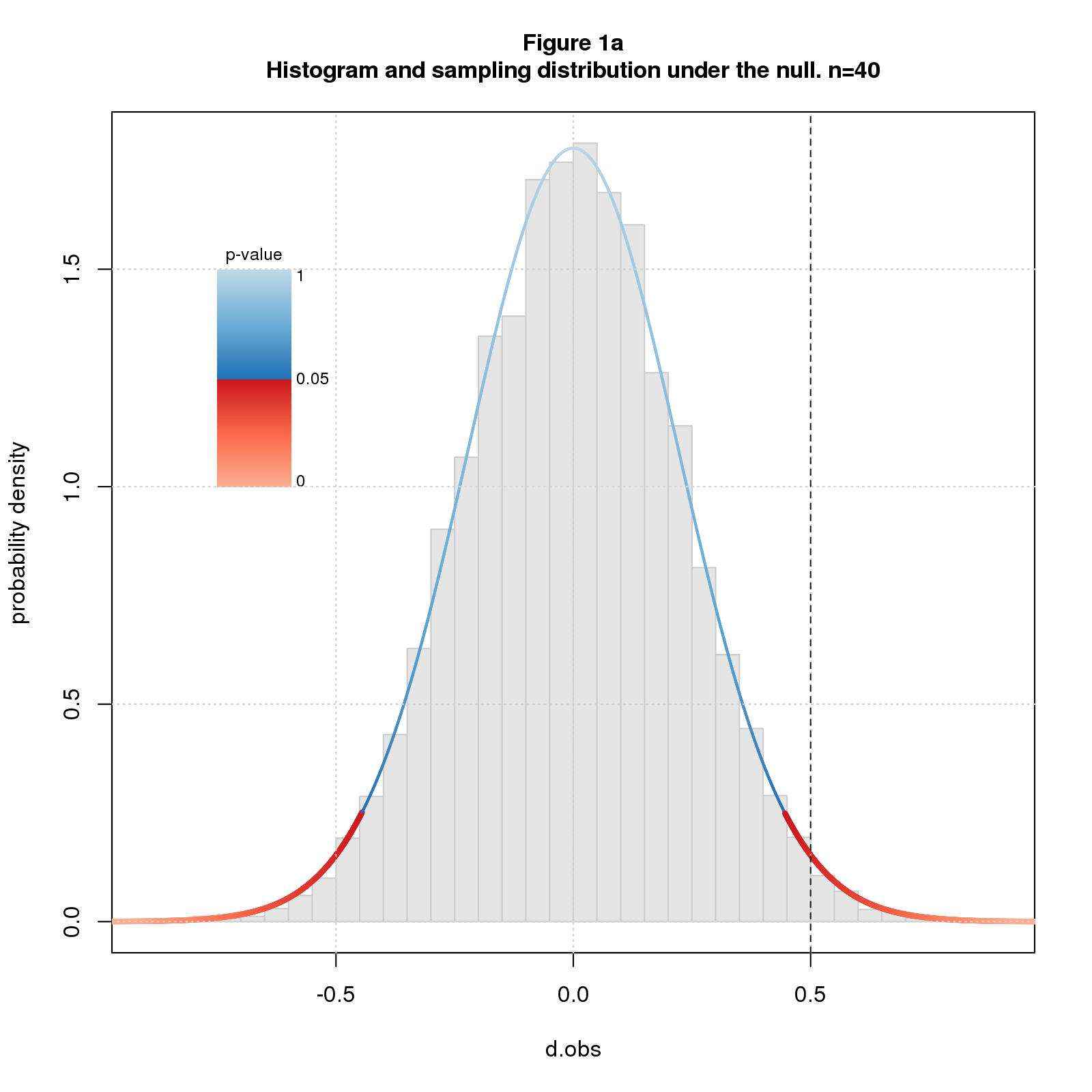Confidence intervals get top billing as the alternative to significance. But beware: confidence intervals rely on the same math as significance and share the same shortcominings. Confidence intervals don’t tell where the true effect lies even probabilistically. What they do is delimit a range of true effects that are broadly consistent with the observed effect.

Confidence intervals, like p-values and power, imagine we’re repeating a study an infinite number of times, drawing a different sample each time from the same population. Though unnatural for basic, exploratory research, it’s a useful mathematical trick that let’s us define the concept of sampling distribution - the distribution of expected results - which in turn is the basis for many common stats. The math is the same across the board; I’ll start with a pedantic explanation of p-values, then generalize the terminology a bit, and use the new terminology to explain confidence intervals.

Recall that the (two-sided) p-value for an observed effect \(d_{obs}\) is the probability of getting a result as or more extreme than \(d_{obs}\) under the null. “Under the null” means we assume the population effect size \(d_{pop}=0\). In math terms, the p-value for \(d_{obs}\) is the tail probability of the sampling distribution - the area under the curve beyond \(d_{obs}\) - times \(2\) to account for the two sides. Recall further that we declare a result to be significant and reject the null when the tail probability is so low that we deem it implausible that \(d_{obs}\) came from the null sampling distribution.

Figure 1a shows a histogram of simulated data overlaid with the sampling distribution for sample size \(n=40\) and \(d_{pop}=0\). I color the sampling distribution by p-value, switching from blue to red at the conventional significance cutoff of \(p=0.05\). The studies are simple two group difference-of-mean studies with equal sample size and standard deviation, and the effect size statistic is standardized difference (aka Cohen’s d). The line at \(d_{obs}=0.5\) falls in the red indicating that we deem the null hypothesis implausible and reject it.